MATLAB Function Referencebvpset

Create or alter boundary value problem (BVP) options structure

Syntax

• ```options = bvpset('name1',value1,'name2',value2,...)
options = bvpset(oldopts'name1',value1,...)
options = bvpset(oldopts,newopts)
bvpset
```

Description

```options = bvpset('name1',value1,'name2',value2,...) ``` creates a structure `options` that you can supply to the boundary value problem solver `bvp4c`, in which the named properties have the specified values. Any unspecified properties retain their default values. For all properties, it is sufficient to type only the leading characters that uniquely identify the property. `bvpset` ignores case for property names.

```options = bvpset(oldopts,'name1',value1,...) ``` alters an existing options structure `oldopts`. This overwrites any values in `oldopts` that are specified using name/value pairs and returns the modified structure as the output argument.

```options = bvpset(oldopts,newopts) ``` combines an existing options structure `oldopts` with a new options structure `newopts`. Any values set in `newopts` overwrite the corresponding values in `oldopts`.

```bvpset ``` with no input arguments displays all property names and their possible values, indicating defaults with braces `{}`.

You can use the function `bvpget` to query the `options` structure for the value of a specific property.

BVP Properties

`bvpset` enables you to specify properties for the boundary value problem solver `bvp4c`. There are several categories of properties that you can set:

Error Tolerance Properties

Because `bvp4c` uses a collocation formula, the numerical solution is based on a mesh of points at which the collocation equations are satisfied. Mesh selection and error control are based on the residual of this solution, such that the computed solution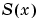is the exact solution of a perturbed problem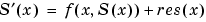. On each subinterval of the mesh, a norm of the residual in the `i`th component of the solution, `res(i)`, is estimated and is required to be less than or equal to a tolerance. This tolerance is a function of the relative and absolute tolerances, `RelTol` and `AbsTol`, defined by the user.

•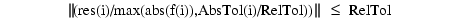The following table describes the error tolerance properties.

 Property Value Description `RelTol` Positive scalar {`1e-3`} A relative error tolerance that applies to all components of the residual vector. It is a measure of the residual relative to the size of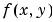. The default, `1e-3`, corresponds to 0.1% accuracy.The computed solutionis the exact solution of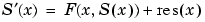. On each subinterval of the mesh, the residual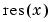satisfies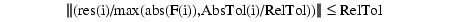`AbsTol` Positive scalar or vector {`1e-6`} Absolute error tolerances that apply to the corresponding components of the residual vector. `AbsTol(i)` is a threshold below which the values of the corresponding components are unimportant. If a scalar value is specified, it applies to all components.

Vectorization

The following table describes the BVP vectorization property. Vectorization of the ODE function used by `bvp4c` differs from the vectorization used by the ODE solvers:

• For `bvp4c`, the ODE function must be vectorized with respect to the first argument as well as the second one, so that `F([x1 x2 ...],[y1 y2 ...])` returns `[F(x1,y1) F(x2,y2) ...]`.
• `bvp4c` benefits from vectorization even when analytical Jacobians are provided. For stiff ODE solvers, vectorization is ignored when analytical Jacobians are used.

 Property Value Description `Vectorized` `on` | {`off`} Set `on` to inform `bvp4c` that you have coded the ODE function `F` so that `F([x1 x2 ...],[y1 y2 ...])` returns `[F(x1,y1) F(x2,y2) ...]`. That is, your ODE function can pass to the solver a whole array of column vectors at once. This enables the solver to reduce the number of function evaluations and may significantly reduce solution time.With the MATLAB array notation, it is typically an easy matter to vectorize an ODE function. In the `shockbvp` example shown previously, the `shockODE` function has been vectorized using colon notation into the subscripts and by using the array multiplication (`.*`) operator.```function dydx = shockODE(x,y,e) pix = pi*x; dydx = [ y(2,:) -x/e.*y(2,:)-pi^2*cos(pix)-pix/e.*sin(pix) ]; ```

Analytical Partial Derivatives

By default, the `bvp4c` solver approximates all partial derivatives with finite differences. `bvp4c` can be more efficient if you provide analytical partial derivatives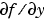of the differential equations, and analytical partial derivatives,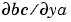and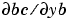, of the boundary conditions. If the problem involves unknown parameters, you must also provide partial derivatives,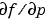and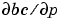, with respect to the parameters.

The following table describes the analytical partial derivatives properties.

 Property Value Description `FJacobian` Function handle Handle to a function that computes the analytical partial derivatives of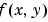. When solving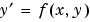, set this property to `@fjac` if `dfdy = fjac(x,y)` evaluates the Jacobian. If the problem involves unknown parameters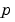, `[dfdy,dfdp] = fjac(x,y,p)` must also return the partial derivative. For problems with constant partial derivatives, set this property to the value of `dfdy` or to a cell array `{dfdy,dfdp}`.See Function Handles in the MATLAB Programming documentation for more information. `BCJacobian` Function handle Handle to a function that computes the analytical partial derivatives of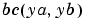. For boundary conditions, set this property to `@bcjac` if [`dbcdya,dbcdyb] = bcjac(ya,yb)` evaluates the partial derivatives, and. If the problem involves unknown parameters, `[dbcdya,dbcdyb,dbcdp] = bcjac(ya,yb,p)` must also return the partial derivative. For problems with constant partial derivatives, set this property to a cell array `{dbcdya,dbcdyb}` or `{dbcdya,dbcdyb,dbcdp}`.

Singular BVPs

`bvp4c` can solve singular problems of the form

•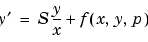posed on the interval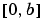where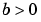. For such problems, specify the constant matrix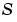as the value of `SingularTerm`. For equations of this form, `odefun` evaluates only the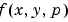term, whererepresents unknown parameters, if any.

 Property Value Description `SingularTerm` Constant matrix Singular term of singular BVPs. Set to the constant matrixfor equations of the formposed on the intervalwhere.

Mesh Size Property

`bvp4c` solves a system of algebraic equations to determine the numerical solution to a BVP at each of the mesh points. The size of the algebraic system depends on the number of differential equations (`n`) and the number of mesh points in the current mesh (`N`). When the allowed number of mesh points is exhausted, the computation stops, `bvp4c` displays a warning message and returns the solution it found so far. This solution does not satisfy the error tolerance, but it may provide an excellent initial guess for computations restarted with relaxed error tolerances or an increased value of `NMax`.

The following table describes the mesh size property.

 Property Value Description `NMax` positive integer {`floor(1000/n)}` Maximum number of mesh points allowed when solving the BVP, where `n` is the number of differential equations in the problem. The default value of `NMax` limits the size of the algebraic system to about 1000 equations. For systems of a few differential equations, the default value of `NMax` should be sufficient to obtain an accurate solution.

Solution Statistic Property

The `Stats` property lets you view solution statistics.

The following table describes the solution statistics property.

 Property Value Description `Stats` `on` | {`off`} Specifies whether statistics about the computations are displayed. If the `stats` property is `on`, after solving the problem, `bvp4c` displays: The number of points in the mesh The maximum residual of the solution The number of times it called the differential equation function `odefun` to evaluateThe number of times it called the boundary condition function `bcfun` to evaluate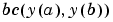Example

To create an options structure that changes the relative error tolerance of `bvp4c` from the default value of `1e-3` to `1e-4`, enter

• ```options = bvpset('RelTol', 1e-4);
```

To recover the value of `'RelTol'` from `options`, enter

• ```bvpget(options, 'RelTol')

ans =

1.0000e-004
```

See Also

`@` (`function_handle`), `bvp4c`, `bvpget`, `bvpinit`, `deval`

© 1994-2005 The MathWorks, Inc.